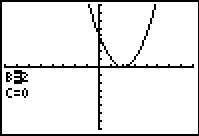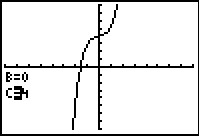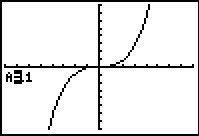••• ##### Device
• TI-83 Plus Family
• TI-84 Plus
• TI-84 Plus Silver Edition
•TI-84 Plus C Silver Edition
•TI-84 Plus CE
• ##### Software

TI Connect™
TI Connect™ CE

# Precalculus: Just Move It

by Texas Instruments#### Overview

Students explore translations, compressions, and stretches of the parent function graph f(x) = x2 and f(x) = x3.

#### Key Steps

•In this activity, students explore transformations of graphs. Modifications are made to the input and output of parent functions. In the first problem, they will begin with the transformation of f(x) → f(x–h), by changing the input values.

•Students will then complete the transformation of ff(x)→f(x)+k, by changing the output values. They will confirm their observations by exploring the transformation of h and k together.

•If time permits, students can explore the transformation of ff(x)→af(x). They will use fractions as well as whole numbers in their investigation. Students will also compare negative a-values to positive a-values.